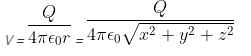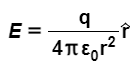# Problem: The potential due to a point charge Q at the origin may be written as Part A. Calculate Ex using equation Ex=−∂V/∂x.Express your answer in terms of the given quantities and appropriate constants.Part B. Calculate Ey using equation Ey=−∂V/∂y.Express your answer in terms of the given quantities and appropriate constants.Part C. Calculate Ez using equation Ez=−∂V/∂z.Express your answer in terms of the given quantities and appropriate constants.Part D. Show that the results of parts (a), (b), (c) agree with the equation for the electric field of a point charge

###### FREE Expert Solution

The electric field is given by:

$\overline{){\mathbf{E}}{\mathbf{=}}{\mathbf{-}}{\mathbf{∆}}{\mathbf{V}}}$

79% (193 ratings)###### Problem Details

The potential due to a point charge Q at the origin may be written asPart A. Calculate Ex using equation Ex=−∂V/∂x.
Express your answer in terms of the given quantities and appropriate constants.
Part B. Calculate Ey using equation Ey=−∂V/∂y.
Express your answer in terms of the given quantities and appropriate constants.
Part C. Calculate Ez using equation Ez=−∂V/∂z.
Express your answer in terms of the given quantities and appropriate constants.
Part D. Show that the results of parts (a), (b), (c) agree with the equation for the electric field of a point chargeFrequently Asked Questions

What scientific concept do you need to know in order to solve this problem?

Our tutors have indicated that to solve this problem you will need to apply the Electric Potential Energy concept. You can view video lessons to learn Electric Potential Energy. Or if you need more Electric Potential Energy practice, you can also practice Electric Potential Energy practice problems.

What professor is this problem relevant for?

Based on our data, we think this problem is relevant for Professor Johnson's class at Maricopa Community Colleges - South Mountain Community College.